• ## 最小费用流

千次阅读 2016-07-19 20:16:14
* 最小费用流 O(V * E * f) * INIT: network g; g.build(v, e); * CALL: g.mincost(s, t); flow=g.flow; cost=g.cost; * 注意: SPFA增广, 实际复杂度远远小于O(V * E); */ #define typef int
ACM模版
O(V * E * f)
/*
*  最小费用流 O(V * E * f)
*  INIT: network g; g.build(v, e);
*  CALL: g.mincost(s, t); flow=g.flow; cost=g.cost;
*  注意: SPFA增广, 实际复杂度远远小于O(V * E);
*/
#define typef int               //  type of flow
#define typec int               //  type of dis
const typef inff = 0x3f3f3f3f;  //  max of flow
const typec infc = 0x3f3f3f3f;  //  max of dis
const int E = 10010;
const int N = 1010;

struct network
{
int nv, ne, pnt[E], nxt[E];
int vis[N], que[N], head[N], pv[N], pe[N];
typef flow, cap[E];
typec cost, dis[E], d[N];
void addedge(int u, int v, typef c, typec w)
{
pnt[ne] = v;
cap[ne] = c;
dis[ne] = +w;
pnt[ne] = u;
cap[ne] = 0;
dis[ne] = -w;
}
int mincost(int src, int sink)
{
int i, k, f, r;
typef mxf;
for (flow = 0, cost = 0; ;)
{
memset(pv, -1, sizeof(pv));
memset(vis, 0, sizeof(vis));
for (i = 0; i < nv; ++i)
{
d[i] = infc;
}
d[src] = 0;
pv[src] = src;
vis[src] = 1;
for (f = 0, r = 1, que = src; r != f;)
{
i = que[f++];
vis[i] = 0;
if (N == f)
{
f = 0;
}
for (k = head[i]; k != -1; k = nxt[k])
{
if(cap[k] && dis[k]+d[i] < d[pnt[k]])
{
d[pnt[k]] = dis[k] + d[i];
if (0 == vis[pnt[k]])
{
vis[pnt[k]] = 1;
que[r++] = pnt[k];
if (N == r)
{
r = 0;
}
}
pv[pnt[k]] = i;
pe[pnt[k]] = k;
}
}
}
if (-1 == pv[sink])
{
break;
}
for (k = sink, mxf = inff; k != src; k = pv[k])
{
if (cap[pe[k]] < mxf)
{
mxf = cap[pe[k]];
}
}
flow += mxf;
cost += d[sink] * mxf;
for (k = sink; k != src; k = pv[k])
{
cap[pe[k]] -= mxf;
cap[pe[k] ^ 1] += mxf;
}
}
return cost;
}

void build(int v, int e)
{
nv = v;
ne = 0;
int x, y;
typef f;
typec w;
for (int i = 0; i < e; ++i)
{
cin >> x >> y >> f >> w;    //  vertex: 0 ~ n-1
}
}
} g;
O(V^2 * f)
/*
*  最小费用流 O(V^2 * f)
*  INIT: network g; g.build(nv, ne);
*  CALL: g.mincost(s, t); flow=g.flow; cost=g.cost;
*  注意: 网络中弧的cost需为非负. 若存在负权, 进行如下转化:
*  首先如果原图有负环, 则不存在最小费用流. 那么可以用Johnson
*  重标号技术把所有边变成正权，以后每次增广后进行维护，算法如下：
*  1、用bellman-ford求s到各点的距离phi[];
*  2、以后每求一次最短路，设s到各点的最短距离为dis[];
*      for i = 1 to v do
*          phi[v] += dis[v];
*  下面的代码已经做了第二步，如果原图有负权，添加第一步即可。
*/
#define typef int               //  type of flow
#define typec int               //  type of cost
const typef inff = 0x3f3f3f3f;  //  max of flow
const typec infc = 0x3f3f3f3f;  //  max of cost
const int E = 10010;
const int N = 1010;

struct edge
{
int u, v;
typef cuv, cvu, flow;
typec cost;
edge (int x, int y, typef cu, typef cv, typec cc) :u(x), v(y), cuv(cu), cvu(cv), flow(0), cost(cc){}
int other(int p)
{
return p == u ? v : u;
}
typef cap(int p)
{
return p == u ? cuv-flow : cvu+flow;
}
typec ecost(int p)
{
if (flow == 0)
{
return cost;
}
else if (flow > 0)
{
return p == u ? cost : -cost;
}
else
{
return p == u ? -cost : cost;
}
}
{
flow += (p == u ? f : -f);
}
};

struct network
{
vector<edge> eg;
vector<edge*> net[N];
edge *prev[N];
int v, s, t, pre[N], vis[N];
typef flow;
typec cost, dis[N], phi[N];
bool dijkstra();
void build(int nv, int ne);
typec mincost(int, int);
};

bool network::dijkstra()
{
//  使用O(E * logV)的Dij可降低整体复杂度至 O(E * logV * f)
int i, j, p, u = 0;
typec md, cw;
for (i = 0; i < v; i++)
{
dis[i] = infc;
}
dis[s] = 0;
prev[s] = 0;
pre[s] = -1;
memset(vis, 0, v * sizeof(int));
for (i = 1; i < v; i++)
{
for (md = infc, j = 0; j < v; j++)
{
if (!vis[j] && md > dis[j])
{
md = dis[j];
u = j;
}
}
if (md == infc)
{
break;
}
for (vis[u] = 1, j = (int)net[u].size() - 1; j >= 0; j--)
{
edge *ce = net[u][j];
if (ce->cap(u) > 0)
{
p = ce->other(u);
cw = ce->ecost(u) + phi[u] - phi[p];
//  !!  assert(cw >= 0);
if (dis[p] > dis[u] + cw)
{
dis[p] = dis[u] + cw;
prev[p] = ce;
pre[p] = u;
}
}
}
}
return infc != dis[t];
}

typec network::mincost(int ss, int tt)
{
s = ss;
t = tt;
int i, c;
typef ex;
flow = cost = 0;
memset(phi, 0, sizeof(phi));
//  !!  若原图含有负消费的边, 在此处运行Bellmanford
//  将phi[i](0 <= i <= n - 1)置为mindist(s, i).
for (i = 0; i < v; i++)
{
net[i].clear();
}
for (i = (int)eg.size() - 1; i >= 0; i--)
{
net[eg[i].u].push_back(&eg[i]);
net[eg[i].v].push_back(&eg[i]);
}
while (dijkstra())
{
for (ex = inff, c = t; c != s; c = pre[c])
{
if (ex > prev[c]->cap(pre[c]))
{
ex = prev[c]->cap(pre[c]);
}
}
for (c = t; c != s; c = pre[c])
{
}
flow += ex;
cost += ex * (dis[t] + phi[t]);
for (i = 0; i < v; i++)
{
phi[i] += dis[i];}
}
return cost;
}

void network::build(int nv, int ne)
{
eg.clear();
v = nv;
int x, y;
typef f;
typec c;
for (int i = 0; i < ne; ++i)
{
cin >> x >> y >> f >> c;
eg.push_back(edge(x, y, f, 0, c));
}
return ;
}
展开全文• 最小费用流的最小费用路算法，运算正确返回0，若有负圈返回其中的一个节点
• 最大流，最小费用流，着色的matlab以及Lingo的实现
• 最小费用流问题，网络分析，最大流量最小费用流问题， 支撑树等一些列方法与例题。
• 最小费用流的消圈算法，判断一个费用流是否最优的消圈算法
• 研究了虚拟网络映射动态过程，发现虚拟网络映射代价收益动态倒置现象，提出虚拟网络多路径链路映射的最小费用流模型及算法，适用于在大规模底层网络上在线创建虚拟网络。仿真结果表明本文所提算法能够提高虚拟网络...虚拟网络 网络虚拟化
• MATLAB源码集锦-基于Busacker-Gowan迭代算法最小费用流代码
• 在不同解包裹算法中,最小费用流(MCF)解包裹法可以限制残差点误差远程扩散,并将误差优先限制在低相干区域,有利于保证高相干区域解包裹结果不受干扰,精度较高,但残差点数量较多时计算效率很低。为缩短解包裹时间,提出...
• 最近小刷了一下最小费用流，久违的小结一下。 最小费用流常见的有两种算法： 一种是常用的连续最短路算法。 另一种是消负圈算法（任意求出一个流，然后不断消去负圈而得到的最小费用流），这种只看到poj 2175 有...
最近小刷了一下最小费用流，久违的小结一下。
最小费用流常见的有两种算法：
一种是常用的连续最短路算法。
另一种是消负圈算法（任意求出一个流，然后不断消去负圈而得到的最小费用流），这种只看到poj 2175 有相关的应用，而且只是求次优，求完整的最小费用流还是第一种好。
最小费用流中，模板都是固定的，关键在于如何建图。
建图过程在于如何将问题如何抽象出来，最小费用流只有两个特点：一是费用，二是流量;
流量一般是将题目中的限制条件进行抽象（比如只能（或者做多）通过k次），这种情况下只要连一条流量为k的边就好。
拆点是最小费用流里特别常见的一种建图手段。我所知的拆点一般有以下几种情况：
1.将点的权值作为费用的时候，题目要求点只能过一次，此时需将一个点拆成两个点，流量为1.
2.同一个点需要与不同性质的点连接的时候. 
展开全文• 本文研究了无容量限制的带固定费用和可变费用的单物资和二物资的最小费用流问题,并分别给出了多项式算法。最后应用该算法,计算了一个二物资的最小费用流问题的实例。
• 针对目标跟踪中的物体遮挡、光照影响、杂波扰动等问题,设计一种基于最小费用流建模的跟踪器.该跟踪器把整数规划与最小费用流模型相结合,将目标跟踪问题转变为可解的线性规划问题.与其他同类型跟踪器相比,该跟踪器...
• 为了运用蚁群算法解决最小费用流问题,首先结合有向网络描述了最小费用流数学模型,运用从终点向始点反向计算的思想求解在最大可行流约束下的最小费用,然后给出了其具体过程。最后通过仿真实验,调整圈法和标号算法验证...
• 最小费用流的允许边算法运用于运输问题,提出了求解运输问题的一种新解法。构造运输问题的最小费用最大流模型,并用允许边算法求得容量-费用网络的最小费用最大流,此最大流对应于运输问题的最优调运方案。在迭代过程...
• 方便初学者的程序，易懂简单，最小费用流的matlab 程序，希望有需要者前来
• 基于Busacker-Gowan迭代算法最小费用流matlab代码.zipmatlab
• 传统的交通网络最小费用流分配是针对单一品种,但在实际的交通运输应用中,交通网络中往往会出现多品种流的运送情况,而且也有可能对某些品种的运送路径进行限制.首先针对交通网络中的多品种流及其流动现象进行分析,...
• ## 最小费用流及其求法

万次阅读 多人点赞 2019-05-04 08:33:48
【1】图与网络模型及方法：图与网络的基本概念 【2】图&网络模型应用—最短路径问题 【3】树：基本概念与最小生成树 ...【7】最小费用流及其求法 ： 【8】最大流问题 【10】钢管订购和运输问题 目录...

【1】图与网络模型及方法：图与网络的基本概念
【2】图&网络模型应用—最短路径问题
【3】树：基本概念与最小生成树
【4】匹配问题： 匈牙利算法 、最优指派、相等子图
【5】Euler 图和 Hamilton 图
【6】计划评审方法和关键路线法【统筹方法】：广泛地用于系统分析和项 目管理
【7】最小费用流及其求法 ：
【8】最大流问题
【10】钢管订购和运输问题

目录
1 最小费用流                         最小费用流问题的线性规划表示                例 19（最小费用最大流问题）
2 求最小费用流的一种方法—迭代法

1 最小费用流
上面我们介绍了一个网络上最短路以及最大流的算法，但是还没有考虑到网络上流 的费用问题，在许多实际问题中，费用的因素很重要。例如，在运输问题中，人们总是 希望在完成运输任务的同时，寻求一个使总的运输费用最小的运输方案。这就是下面要 介绍的最小费用流问题。
最小费用流问题的线性规划表示
在运输网络 N = (s,t,V, A,U) 中，设  是定义在 A 上的非负函数，它表示通过弧 (i, j) 单位流的费用。所谓最小费用流问题就是从发点到收点怎样以最小费用输送一已 知量为v( f ) 的总流量。 最小费用流问题可以用如下的线性规划问题描述：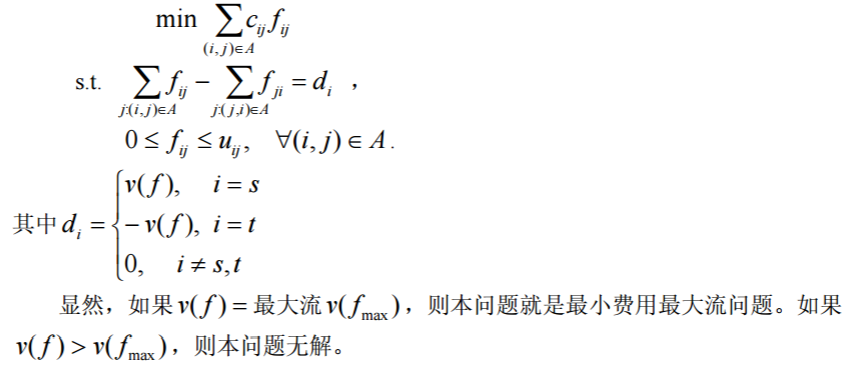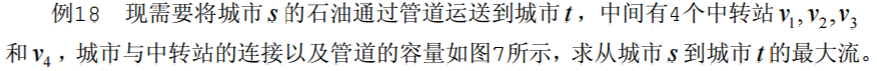例 19（最小费用最大流问题）
（续例 18）由于输油管道的长短不一或地质等原因， 使每条管道上运输费用也不相同，因此，除考虑输油管道的最大流外，还需要考虑输油 管道输送最大流的最小费用。图 8 所示是带有运费的网络，其中第 1 个数字是网络的容 量，第 2 个数字是网络的单位运费。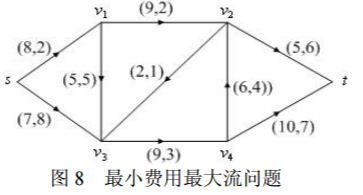解 按照最小费用流的数学规划写出相应的 LINGO 程序如下：
model:
sets:
nodes/s,1,2,3,4,t/:d;
arcs(nodes,nodes)/s 1,s 3,1 2,1 3,2 3,2 t,3 4,4 2,4 t/:c,u,f;
endsets
data:
d=14 0 0 0 0 -14; !最大流为14;
c=2 8 2 5 1 6 3 4 7;
u=8 7 9 5 2 5 9 6 10;
enddata
min=@sum(arcs:c*f);
@for(nodes(i):@sum(arcs(i,j):f(i,j))-@sum(arcs(j,i):f(j,i))=d(i));
@for(arcs:@bnd(0,f,u));
end
求得最大流的最小费用是 205，而原最大流的费用为 210 单位，原方案并不是最优 的。 类似地，可以利用赋权邻接矩阵编程求得最小费用最大流。LINGO 程序如下：
model:
sets:
nodes/s,1,2,3,4,t/:d;
arcs(nodes,nodes):c,u,f;
endsets
data:
d=14 0 0 0 0 -14;
c=0; u=0;
enddata
calc:
c(1,2)=2;c(1,4)=8;
c(2,3)=2;c(2,4)=5;
c(3,4)=1;c(3,6)=6;
c(4,5)=3;c(5,3)=4;c(5,6)=7;
u(1,2)=8;u(1,4)=7;
u(2,3)=9;u(2,4)=5;
u(3,4)=2;u(3,6)=5;
u(4,5)=9;u(5,3)=6;u(5,6)=10;
endcalc
min=@sum(arcs:c*f);
@for(nodes(i):@sum(nodes(j):f(i,j))-@sum(nodes(j):f(j,i))=d(i));
@for(arcs:@bnd(0,f,u));
end 
2 求最小费用流的一种方法—迭代法
这里所介绍的求最小费用流的方法叫做迭代法。这个方法是由 Busacker 和 Gowan 在 1961 年提出的。其主要步骤如下：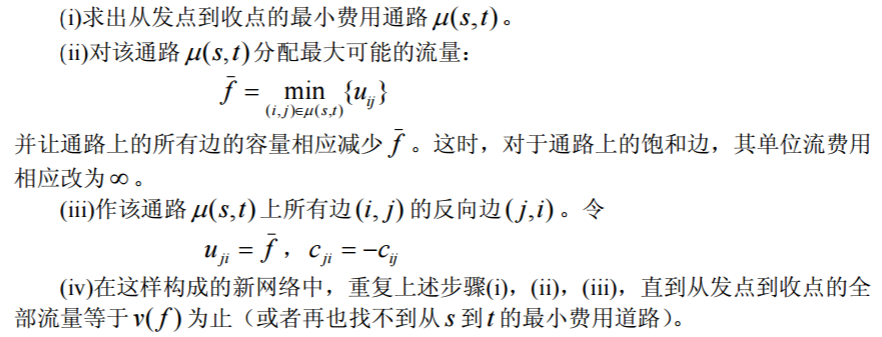下面我们编写了最小费用最大流函数 mincostmaxflow，其中调用了利用 Floyd 算法 求最短路的函数 floydpath。
求解例 19 具体程序如下（下面的全部程序放在一个文件中）：
function mainexample19
clear;clc;
global M num
c=zeros(6);u=zeros(6);
c(1,2)=2;c(1,4)=8;c(2,3)=2;c(2,4)=5;
c(3,4)=1;c(3,6)=6;c(4,5)=3;c(5,3)=4;c(5,6)=7;
u(1,2)=8;u(1,4)=7;u(2,3)=9;u(2,4)=5;
u(3,4)=2;u(3,6)=5;u(4,5)=9;u(5,3)=6;u(5,6)=10;
num=size(u,1);M=sum(sum(u))*num^2;
[f,val]=mincostmaxflow(u,c)
%求最短路径函数
function path=floydpath(w);
global M num
w=w+((w==0)-eye(num))*M;
p=zeros(num);
for k=1:num
for i=1:num
for j=1:num
if w(i,j)>w(i,k)+w(k,j)
w(i,j)=w(i,k)+w(k,j);
p(i,j)=k;
end
end
end
end
if w(1,num) ==M
path=[];
else
path=zeros(num);
s=1;t=num;m=p(s,t);
while ~isempty(m)
if m(1)
s=[s,m(1)];t=[t,t(1)];t(1)=m(1);
m(1)=[];m=[p(s(1),t(1)),m,p(s(end),t(end))];
else
path(s(1),t(1))=1;s(1)=[];m(1)=[];t(1)=[];
end
end
end
%最小费用最大流函数
function [flow,val]=mincostmaxflow(rongliang,cost,flowvalue);
%第一个参数：容量矩阵；第二个参数：费用矩阵；
%前两个参数必须在不通路处置零
%第三个参数：指定容量值（可以不写，表示求最小费用最大流）
%返回值 flow 为可行流矩阵,val 为最小费用值
global M
flow=zeros(size(rongliang));allflow=sum(flow(1,:));
if nargin<3
flowvalue=M;
end
while allflow<flowvalue
w=(flow<rongliang).*cost-((flow>0).*cost)';
path=floydpath(w);%调用 floydpath 函数
if isempty(path)
val=sum(sum(flow.*cost));
return;
end
theta=min(min(path.*(rongliang-flow)+(path.*(rongliang-flow)==0).*M));
theta=min([min(path'.*flow+(path'.*flow==0).*M),theta]);
flow=flow+(rongliang>0).*(path-path').*theta;
allflow=sum(flow(1,:));
end
val=sum(sum(flow.*cost)); 

【1】图与网络模型及方法：图与网络的基本概念
【2】图&网络模型应用—最短路径问题
【3】树：基本概念与最小生成树
【4】匹配问题： 匈牙利算法 、最优指派、相等子图
【5】Euler 图和 Hamilton 图
【6】计划评审方法和关键路线法【统筹方法】：广泛地用于系统分析和项 目管理
【7】最小费用流及其求法 ：
【8】最大流问题
【10】钢管订购和运输问题

3 图与网络模型习题
1. 一只狼、一头山羊和一箩卷心菜在河的同侧。一个摆渡人要将它们运过河去， 但由于船小，他一次只能运三者之一过河。显然，不管是狼和山羊，还是山羊和卷心菜， 都不能在无人监视的情况下留在一起。问摆渡人应怎样把它们运过河去？
2. 北京（Pe）、东京(T)、纽约(N)、墨西哥城(M)、伦敦(L)、巴黎(Pa)各城市之间的 航线距离如表 16。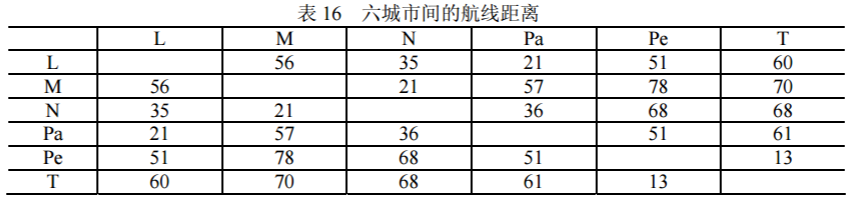由上述交通网络的数据确定最小生成树。
3. 某台机器可连续工作 4 年，也可于每年末卖掉，换一台新的。已知于各年初购 置一台新机器的价格及不同役龄机器年末的的处理价如表 17 所示。又新机器第一年运 行及维修费为 0.3 万元，使用 1-3 年后机器每年的运行及维修费用分别为 0.8，1.5，2.0 万元。试确定该机器的最优更新策略，使 4 年内用于更换、购买及运行维修的总费用为最省。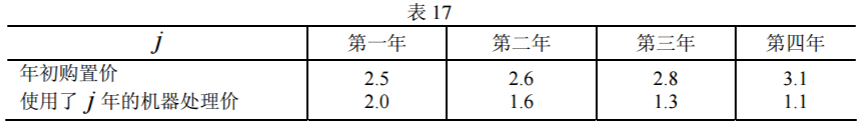4. 某产品从仓库运往市场销售。已知各仓库的可供量、各市场需求量及从i 仓库 至 j 市场的路径的运输能力如表 18 所示（表中数字 0 代表无路可通），试求从仓库可运 往市场的最大流量，各市场需求能否满足？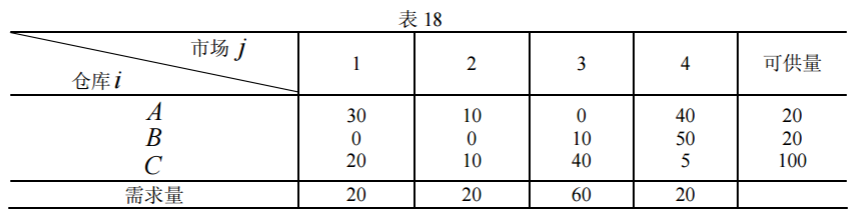5. 某单位招收懂俄、英、日、德、法文的翻译各一人，有 5 人应聘。已知乙懂俄 文，甲、乙、丙、丁懂英文，甲、丙、丁懂日文，乙、戊懂德文，戊懂法文，问这 5 个人是否都能得到聘书？最多几个得到聘书，招聘后每人从事哪一方面翻译工作？
6. 表 19 给出某运输问题的产销平衡表与单位运价表。将此问题转化为最小费用最 大流问题，画出网络图并求数值解。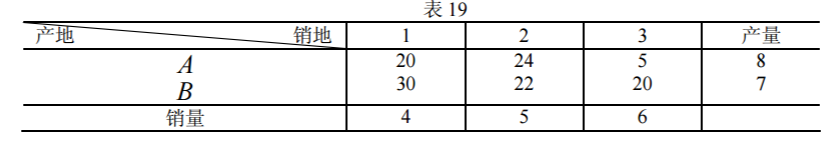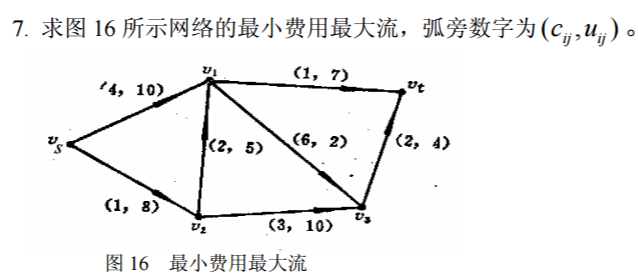8. 某公司计划推出一种新型产品，需要完成的作业由表 20 所示。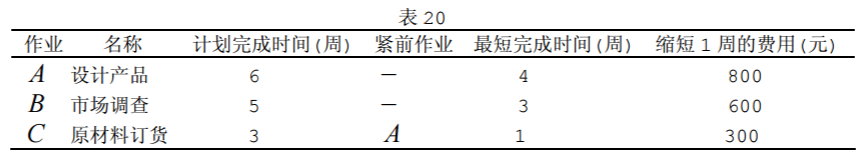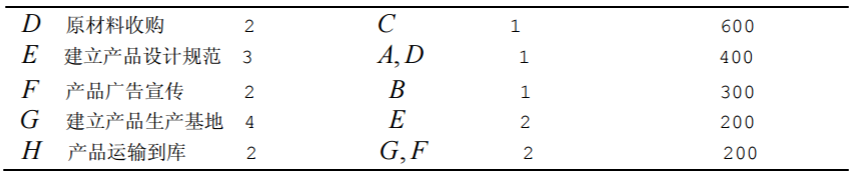（1）画出产品的计划网络图；
（2）求完成新产品的最短时间，列出各项作业的最早开始时间、最迟开始时间和 计划网络的关键路线；
（3）假定公司计划在 17 周内推出该产品，各项作业的最短时间和缩短 1 周的费 用如上表所示，求产品在 17 周内上市的最小费用；
（4）如果各项作业的完成时间并不能完全确定，而是根据以往的经验估计出来的， 其估计值如表 21 所示。试计算出产品在 21 周内上市的概率和以 95％的概率完成新产 品上市所需的周数。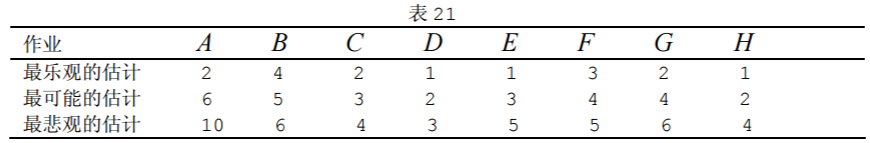9．已知下列网络图有关数据如表 22，设间接费用为 15 元/天，求最低成本日程。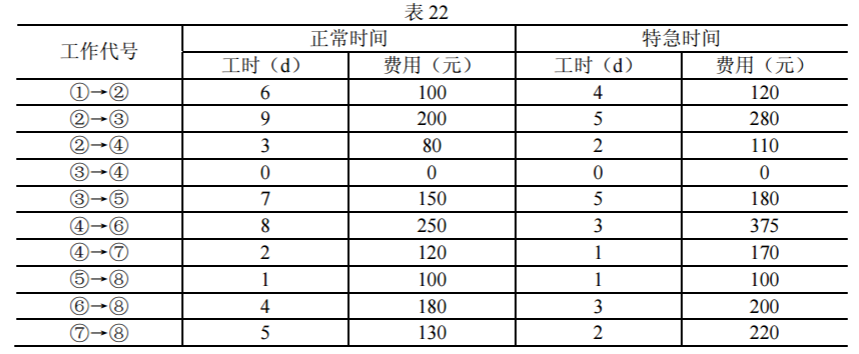展开全文• 论文研究-求解最小费用流的复合标号法.pdf, 本文在求最短路和求最大流标号法的基础上,提出了求最小费用流的复合标号法。这种方法比现在流行的算法简单易行,迭代次数少,而且易于理解和为一般读者所接受。
• ## 最小费用流模板

千次阅读 2016-05-30 18:02:58
和最大流模板对比着看：...贴上最小费用流模板： const int oo=1e9; const int mm=11111111; const int mn=888888; int node,src,dest,edge; int ver[mm],flow[mm],cost[mm],nex[mm]; int head[mn],dis[mn],p[mn],q[mn]
想看更多模板？请点击：http://blog.csdn.net/martinue/article/category/6268283
和最大流模板对比着看：最大流模板（Dinic）
贴上最小费用流模板：

const   int oo=1e9;
const   int mm=11111111;
const   int mn=888888;
int node,src,dest,edge;
int ver[mm],flow[mm],cost[mm],nex[mm];
/**这些变量基本与最大流相同，增加了
cost 表示边的费用，
p 记录可行流上节点对应的反向边
*/
void prepare(int _node,int _src,int _dest)
{
node=_node,src=_src,dest=_dest;
edge=0;
}
void addedge(int u,int v,int f,int c)
{
}
/**以上同最大流*/
/**spfa 求最短路，并用 p 记录最短路上的边*/
bool spfa()
{
int i,u,v,l,r=0,tmp;
for(i=0; i<node; ++i)dis[i]=oo;
dis[q[r++]=src]=0;
p[src]=p[dest]=-1;
for(l=0; l!=r; (++l>=mn)?l=0:l)
if(flow[i]&&dis[v=ver[i]]>(tmp=dis[u]+cost[i]))
{
dis[v]=tmp;
p[v]=i^1;
if(vis[v]) continue;
vis[q[r++]=v]=1;
if(r>=mn)r=0;
}
return p[dest]>-1;
}
/**源点到汇点的一条最短路即可行流，不断的找这样的可行流*/
int SpfaFlow()
{
int i,ret=0,delta;
while(spfa())
{
for(i=p[dest],delta=oo; i>=0; i=p[ver[i]])
if(flow[i^1]<delta)delta=flow[i^1];
for(i=p[dest]; i>=0; i=p[ver[i]])
flow[i]+=delta,flow[i^1]-=delta;
ret+=delta*dis[dest];
}
return ret;
}


展开全文acm
• 基于最小费用流模型的无重叠视域多摄像机目标关联算法研究论文
• ## POJ 2195 最小费用流

千次阅读 2016-04-21 20:41:46
题意：给个乱七八糟的方阵，H代表家，m代表人，现在所有人都要回到一个家，问所有人走到家的步数和 思路：还是很好想到费用流的，费用为人走到家的...跑最小费用流就是结果了，PS：入门题，还是蛮简单的.........#inACM poj
• 论文研究-最小费用流在商品购运销中的应用.pdf, 本文力图用网络技术中的最小费用流理论解决商品流通企业降低其商品购、运、销中的成本问题。
• 最小费用流问题，可视为一般化的最短路径问题和最大流问题，即只要选定合适的权重、容量、流量，解决最小费用流的方法就能用来解决上述问题。另一方面，也意味着解最小费用流问题至少和解最短路径或最大流问题一样难...算法 图算法 Floyd
• 网络游戏-使用最小费用流网络的宽基线双目物体匹配方法.zip...